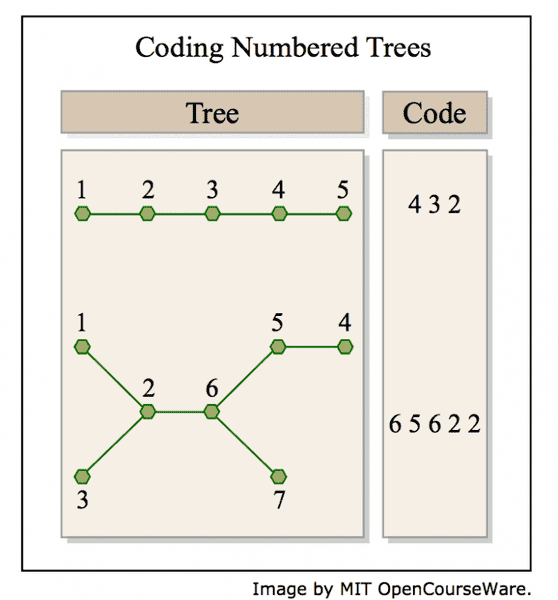# Combinatorics, Assigning Occurrence Numbers to Integers

QuietMind

## Homework Statement

This problem is from MIT OpenCourseWare- a diagram is attached to clarify certain definitions. I'd like to check my answers.

The degree sequence of a simple graph is the weakly decreasing sequence of degrees of its vertices. For example, the degree sequence for the 5-vertex numbered tree pictured in the Figure 1 is (2, 2, 2, 1, 1) and for the 7-vertex tree it is (3, 3, 2, 1, 1, 1, 1). We’re interested in counting how many numbered trees there are with a given degree sequence. We’ll do this using the bijection defined in Problem 16.5 between n-vertex numbered trees and length n − 2 code words whose characters are integers between 1 and n. The occurrence number for a character in a word is the number of times that the character occurs in the word. For example, in the word 65622, the occurrence number for 6 is two, and the occurrence number for 5 is one. The occurrence sequence of a word is the weakly decreasing sequence of occurrence numbers of characters in the word. The occurrence sequence for this word is (2, 2, 1) because it has two occurrences of each of the characters 6 and 2, and one occurrence of 5.

(a) There is simple relationship between the degree sequence of an n-vertex numbered tree and the occurrence sequence of its code. Describe this relationship and explain why it holds. Conclude that counting n-vertex numbered trees with a given degree sequence is the same as counting the number of length n − 2 code words with a given occurrence sequence. Hint: How many times does a vertex of degree, d, occur in the code?For simplicity, let’s focus on counting 9-vertex numbered trees with a given degree sequence. By part (a), this is the same as counting the number of length 7 code words with a given occurrence sequence.

Any length 7 code word has a pattern, which is another length 7 word over the alphabet a,b,c,d,e,f,g that has the same occurrence sequence.

(b) How many length 7 patterns are there with three occurrences of a, two occurrences of b, and one occurrence of c and d?

(c) How many ways are there to assign occurrence numbers to integers 1, 2, . . . , 9 so that a code word with those occurrence numbers would have the occurrence sequence 3, 2, 1, 1, 0, 0, 0, 0, 0?

In general, to find the pattern of a code word, list its characters in decreasing order by number of occurrences, and list characters with the same number of occurrences in decreasing order. Then replace successive characters in the list by successive letters a,b,c,d,e,f,g. The code word 2468751, for example, has the pattern fecabdg, which is obtained by replacing its characters 8,7,6,5,4,2,1 by a,b,c,d,e,f,g, respectively. The code word 2449249 has pattern caabcab, which is obtained by replacing its characters 4,9,2 by a,b,c, respectively.

(d) What length 7 code word has three occurrences of 7, two occurrences of 8, one occurrence each of 2 and 9, and pattern abacbad?

(e) Explain why the number of 9-vertex numbered trees with degree sequence (4, 3, 2, 2, 1, 1, 1, 1, 1) is the product of the answers to parts (b) and (c).

## The Attempt at a Solution

a) The degree sequence tells you the number of degrees each vertex has. In the code, a vertex will be represented by a value that is one less than its degree. So, to go from the degree sequence to occurrence sequence, subtract 1 from each of its elements. If any values are 0 after the subtraction, they are omitted.

b) This is a permutation, I believe. There are 7! total possibilities, but to account for the possible redundancies from the 3 a's and 2 b's, divide total possibilities by 3! and 2! respectively.
##\frac{7!}{3!2!}##

c)
I thought about assigning the integers 1-9 to the various values of the occurrence sequence. This is how I visualized it:

{ _ } { _ } { _ _ } {_ _ _ _ _}
3 2 1's 0's

So of 9! total possibilities for assignment, but divide by a factor of 2! to account for redundancy of the 1's, a factor of 5! to account for redundancy of the 0's

and a factor of 4! to account for redundancy of the permutations of the brackets ie

{ _ } { _ } { _ _ } {_ _ _ _ _}
3 2 1's 0's
vs
{_ _ _ _ _}{ _ } { _ } { _ _ }
0's 3 2 1's
should not be counted as two.
This gives me the result
##\frac{9!}{2!5!4!}##

Is this reasoning correct? b and c are the ones I'm most uncertain about

d) Ordering according to their rules
7,8,9,2
correspond to
a,b,c,d
respectively.
So I get 7879872

e) The answer from part b gives the number of code words possible for each occurrence sequence, and answer from part c gives the number of different ways an occurrence sequence could have its occurrences assigned. Total possibilities is the product.

•